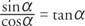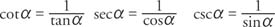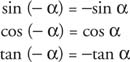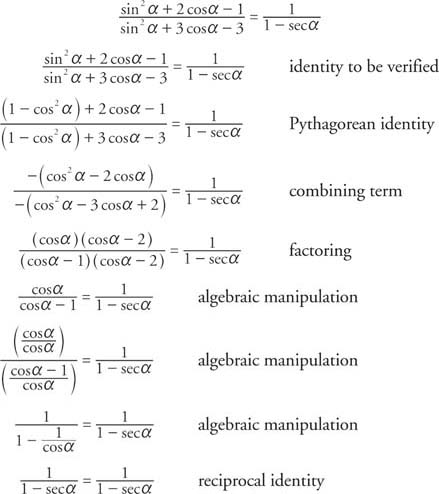## Fundamental Identities

If an equation contains one or more variables and is valid for all replacement values of the variables for which both sides of the equation are defined, then the equation is known as an identity. The equation x 2 + 2 x = x( x + 2), for example, is an identity because it is valid for all replacement values of x.

If an equation is valid only for certain replacement values of the variable, then it is called a conditional equation. The equation 3 x + 4 = 25, for example, is a conditional equation because it is not valid for all replacement values of x. An equation that is said to be an identity without stating any restrictions is, in reality, an identity only for those replacement values for which both sides of the identity are defined. For example, the identityis valid only for those values of α for which both sides of the equation are defined.

The fundamental (basic) trigonometric identities can be divided into several groups. First are the reciprocal identities. These includeNext are the quotient identities. These includeThen there are the cofunction identities. These includeNext there are the identities for negatives. These includeFinally there are the Pythagorean identities. These includeThe second identity is obtained by dividing the first by cos 2 α, and the third identity is obtained by dividing the first by sin 2 α. The process of showing the validity of one identity based on previously known facts is called proving the identity. The validity of the foregoing identities follows directly from the definitions of the basic trigonometric functions and can be used to verify other identities.

No standard method for solving identities exists, but there are some general rules or strategies that can be followed to help guide the process:

1. Try to simplify the more complicated side of the identity until it is identical to the second side of the identity.

2. Try to transform both sides of an identity into an identical third expression.

3. Try to express both sides of the identity in terms of only sines and cosines; then try to make both sides identical.

4. Try to apply the Pythagorean identities as much as possible.

5. Try to use factoring and combining of terms, multiplying one side of the identity by an expression that is equal to 1, squaring both sides of the identity, and other algebraic techniques to manipulate equations.

Example 1: Use the basic trigonometric identities to determine the other five values of the trigonometric

functions given thatExample 2: Verify the identity cos α + sin α tan α = sec α.Example 3: Verify the identityExample 4: Verify the identity